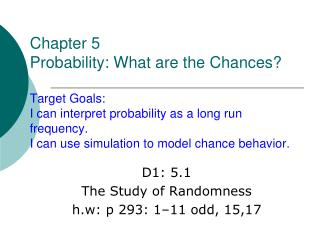# D1: 5.1 The Study of Randomness h.w: p 293: 1–11 odd, 15,17 - PowerPoint PPT PresentationDownload PresentationD1: 5.1 The Study of Randomness h.w: p 293: 1–11 odd, 15,17

D1: 5.1 The Study of Randomness h.w: p 293: 1–11 odd, 15,17
Download Presentation## D1: 5.1 The Study of Randomness h.w: p 293: 1–11 odd, 15,17

- - - - - - - - - - - - - - - - - - - - - - - - - - - E N D - - - - - - - - - - - - - - - - - - - - - - - - - - -
##### Presentation Transcript

1. Chapter 5Probability: What are the Chances?Target Goals:I can interpret probability as a long run frequency.I can use simulation to model chance behavior. D1: 5.1 The Study of Randomness h.w: p 293: 1–11 odd, 15,17

2. Randomness & Probability Models Random? • How would you describe something that is random? Behavior is random if, • while individual outcomes are uncertain • for a large number of repetitions, outcomes are regularly distributed.

3. Randomness Example: • If I roll a die once, I can’t predict with any certainty what number it will land on, but if I roll sixty times, I can expect it to land on 1-ten times, 2-ten times, 3-ten times, etc.

4. Pg. 282

5. Consider flipping a coin and recording the relative frequency of heads. When the number of coin flips is small, there is a lot of variability in the relative frequency of “heads” (as shown in this graph). What do you notice in the graph at the right?

6. The graph at the right shows the relative frequency when the coin is flipped a large number of times. What do you notice in this graph at the right? Consider flipping a coin and recording the relative frequency of heads.

7. Law of Large Numbers Notice how the relative frequency of heads approaches ½ the larger the number of trials!

8. Probability The probability of an outcome • describes the pattern of chance outcome, and • is the proportion of times the outcome would occur for a large number of repetitions. • Example: The probability of a die landing on 4 is the proportion of times a die lands on 4 for a large number of repetitions. OR in other words, after a large number of trials, the relative frequency approaches the true probability.

9. Exercise: SHAQ The basketball player Shaquille O’Neal made about half of his free throws over the entire season. • We will use the calculator to simulate 100 free throws shot independently by a player who has a probability 0.5 of making each shot. • We let the number 1 represent the outcome “Hit” and 0 represent a “Miss”.

10. a. Calculator Keystrokes • Enter the command MATH:PRB:randInt(0, 1, 100) • Store in SHAQ (must press “APHA” key with letter) • This tells the calculator to randomly select a hit (1) or a miss (0), do this 100 times in succession, and store the results in the list named SHAQ.

11. b. What percent of the 100 shots are hits? • Enter STAT: CALC: 1–Var Stats: enter • Retrieve SHAQ from name list. • 2nd STAT(LIST): NAMES • Scroll down to SHAQ: enter • The percent in x bar (mean) records the number of 1’s or “Hits”.

12. c. Examine the sequence of hits and misses. How long was the longest run of shots made? Of shots missed? • Examine SHAQ list: • Clear L1: STAT:ClrList(4): enter: L1 • Store SHAQ in L1

13. The myth of the “law of averages”. • Interesting fact: • sequences of random outcomes often • show runs longer than our intuition thinks likely.

14. Ex: Golden Ticket Parking Lottery Simulation 95 students have permission to park on campus. What is the probability that a fair lottery would result in two winners from the 28 students in the AP Statistics class? Reading across row 139 in Table D, look at pairs of digits until you see two different labels from 01-95. Record whether or not both winners are members of the AP Statistics Class. Based on 18 repetitions of our simulation, both winners came from the AP Statistics class 3 times, so the probability is estimated as 16.67%.

15. Exercise: How Many Tosses to Get a Head? If we toss a penny, what does experience tell you the probability of a head is? Suppose now that we toss the penny repeatedly until we get a head. • What is the probability that the first head comes up in an odd number of tosses (1, 3, 5, and so on)? • To find out, repeat the experiment 50 times, and keep record of the number of tosses needed to get a head on each of your 50 trails.

16. Simulate tossing penny for 50 trails For each trail: • MATH: PROB: randInt (0, 1,1): enter • Press ENTER until a 1 appears. Place a check mark next to trail if it appeared on an odd toss. • Select Enter: for a new trail and repeat 50 times. • Tally results in table provided.

17. Results a. From your experiment, estimate the probability of a head on the first toss. Results will vary. What value should we expect this value to have?

18. b. Estimate the theoretical probability that the first head appears on an odd toss. • P(head on odd # toss) = • ½ + (½)3 + (½)5 + … = 2/3 • Most answers from simulation will be between o.47 and 0.87